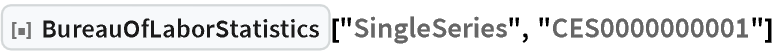# Wolfram CloudResource Object

Function Resource

# BureauOfLaborStatistics

Access data and statistics from the Bureau of Labor Statistics (BLS)

 ResourceFunction["BureauOfLaborStatistics"]["Surveys"] gives a list of all available BLS surveys. ResourceFunction["BureauOfLaborStatistics"]["Survey",survey] gives details about a specific survey. ResourceFunction["BureauOfLaborStatistics"]["PopularSeries"] gives a list of popular BLS data series. ResourceFunction["BureauOfLaborStatistics"]["PopularSeries", survey] give s a list of popular BLS data series for the given survey. ResourceFunction["BureauOfLaborStatistics"]["SingleSeries", id] gives the data series for the given id. ResourceFunction["BureauOfLaborStatistics"]["MultipleSeries", assoc] gives the data series conforming to the assoc specification.

## Details and Options

A survey is a top-level categorization of statistic tracked by the BLS. It is always a two letter code, like "AP", "BD", ….
A series is a survey combined with an alpha-numerical specifier. More details about these specifiers can be found here: https://www.bls.gov/help/hlpforma.htm
In ResourceFunction["BureauOfLaborStatistics"]["Survey", survey] can be any of the surveys returned by ResourceFunction["BureauOfLaborStatistics"]["Surveys"].
Because there are thousands of available data series, the BLS provides a short list of popular and important data series. This can be obtained by evaluating ResourceFunction["BureauOfLaborStatistics"]["PopularSeries"].
Popular data series within a given survey can be obtained with ResourceFunction["BureauOfLaborStatistics"]["PopularSeries", survey].
A single data series can be obtained with ResourceFunction["BureauOfLaborStatistics"]["SingleSeries", id] where id is a series identifier like "CES0000000001".
Complex queries can be obtained ResourceFunction["BureauOfLaborStatistics"]["MultipleSeries", assoc] where assoc has the following keys:
 "seriesid" a list of series ids "startyear" start year (string) of a series "endyear" end year (string) of a series "catalog" whether to include catalog data (boolean) "calculations" whether to include calculations like net and percentage changes (boolean) "annualaverage" whether to include the annual average in the results (boolean) "aspects" whether to include aspects (boolean)

## Examples

### Basic Examples (1)

 In:=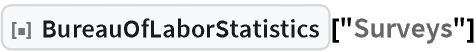Out=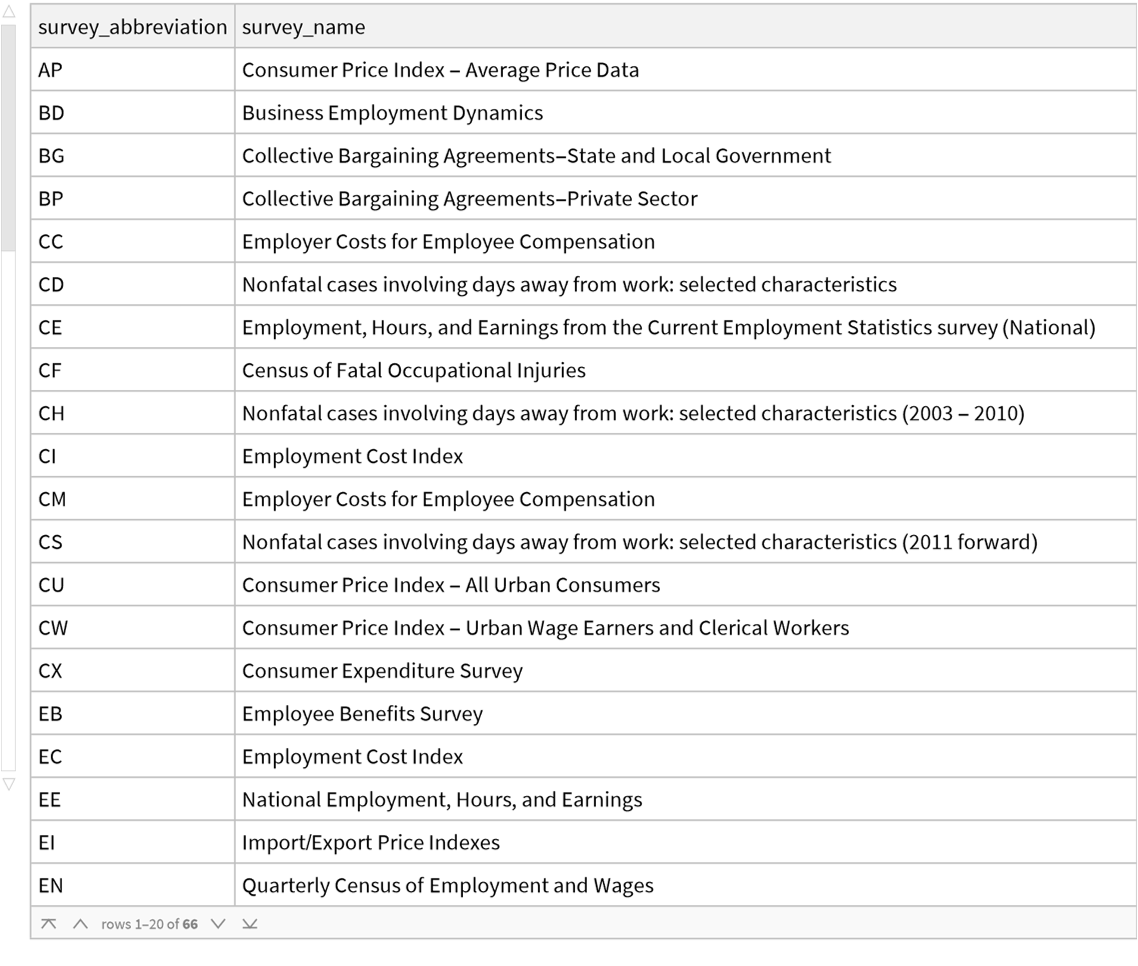In:=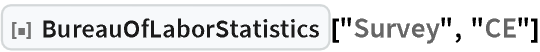Out=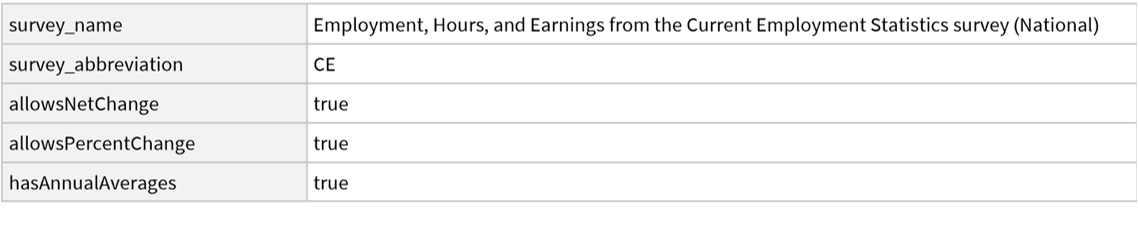In:=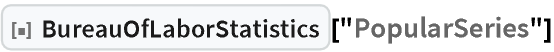Out=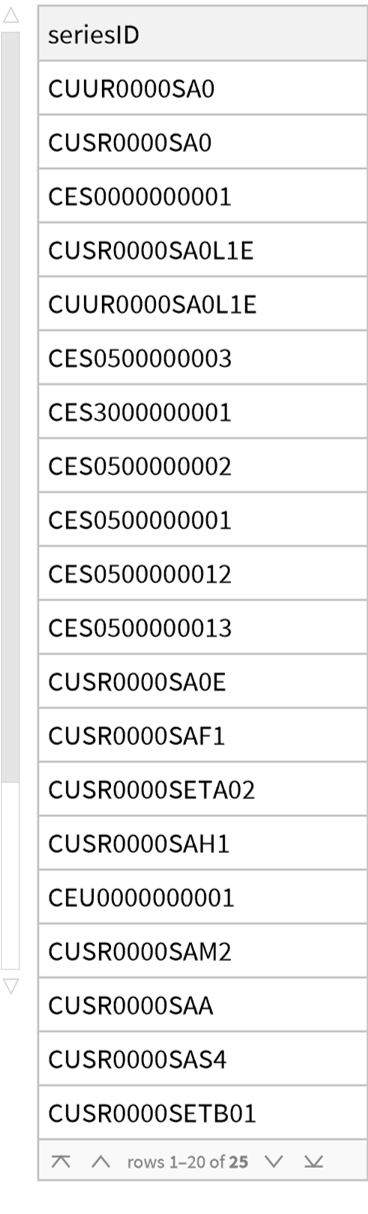In:=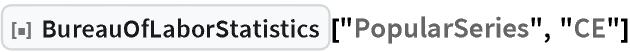Out=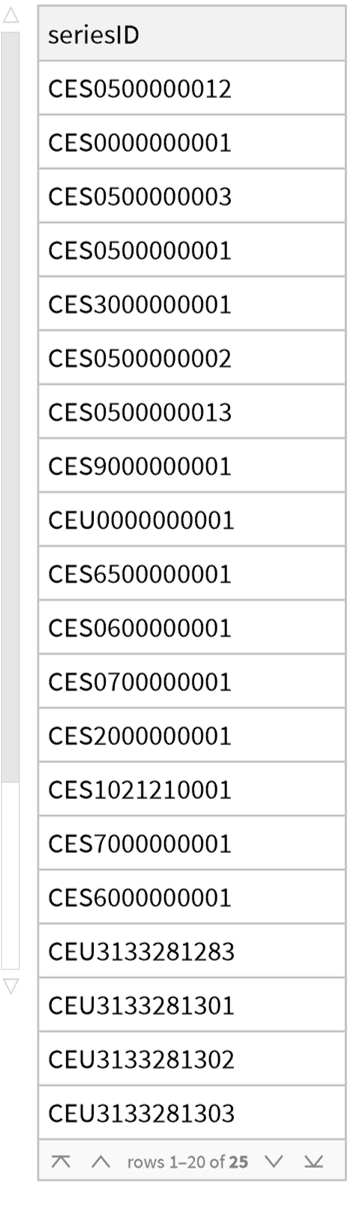In:=